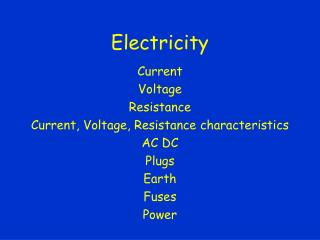DownloadDownload PresentationElectricity

# Electricity

Télécharger la présentation## Electricity

- - - - - - - - - - - - - - - - - - - - - - - - - - - E N D - - - - - - - - - - - - - - - - - - - - - - - - - - -
##### Presentation Transcript

1. Electricity Current Voltage Resistance Current, Voltage, Resistance characteristics AC DC Plugs Earth Fuses Power

2. Current • Current is the amount of charge flowing past a point in a given time Current (A) = Charge (C) / time (s) Current read by an ammeter – connected in series

3. Potential Difference • Voltage • Potential difference is the energy given to or given by a charge when it passes a component. PD (V) = Energy (J) / Charge (C) PD is taken by a voltmeter and is connected in parallel with the component.

4. Resistance • Resistance is the volts per amp for a component. • How many volts are needed to push a current of so many through a component. Resistance (Ohms) = PD (V)/ Current (A)

5. Circuit models • Circuit diagrams • Resistor models

6. IV Characteristics • Resistor • Bulb • Diode

7. Resistors • Thermistor • LDR

8. review

9. Mains Elec • PHET • AC DC • Period and frequency

10. Plugs

11. Fuse • A Fuse is a piece of wire with a low melting point • If there is a fault in the electrical appliance and the current goes up it could damage the wiring in the appliance • But if a fuse is installed, this will melt (or “blow”) and break the circuit • So a fuse is used to protect the appliance – not necessarily to protect us from electric shock

12. Earth • What on Earth does it do? • V d G

13. Earth • No Earth – No Fault

14. Earth • No Earth - Fault

15. Earth • Earth – no Fault

16. Earth • Earth - Fault

17. Power • Power = work done / time taken • Lifting stuff

18. Electrical Power • Electrical power is the electrical work done in a given time. Power (W) = current (A) x voltage (V)